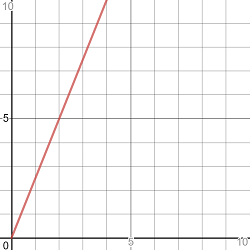## Directly Proportional Relationships

If a quantity {eq}y {/eq} is directly proportional to a quantity {eq}x {/eq}, the relationship between the quantities can be expressed by the equation {eq}y=k\cdot x {/eq} where {eq}k {/eq} is a constant called the constant of proportionality. Instead of saying that {eq}y {/eq} is directly proportional to {eq}x {/eq}, sometimes it is phrased as "{eq}y {/eq} varies directly as {eq}x {/eq}" or "{eq}y {/eq} varies directly with {eq}x {/eq}" or just "{eq}y {/eq} is proportional to {eq}x {/eq}"

Let {eq}y {/eq} represent the total cost and let {eq}x {/eq} represent the number of sandwiches purchased. Since this is a proportional relationship, we can write an equation representing the relationship in the form {eq}y=k\cdot x {/eq} where {eq}k {/eq} is the constant of proportionality. Since we know that 2 sandwiches cost \$5.00, we can solve for the constant of proportionality by substituting these values into our equation.

{eq}y=k\cdot x\\ 5=k\cdot 2\\ \dfrac{5}{2} = k\\ {/eq}

Now that we have the constant of proportionality, we can write the equation representing this particular situation: {eq}y=\dfrac{5}{2} x {/eq}

This equation is a linear equation (a straight line) with a slope of {eq}\dfrac{5}{2} {/eq}